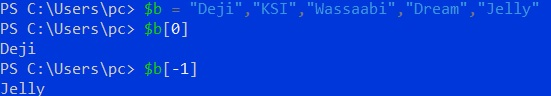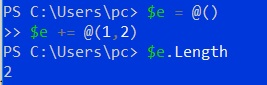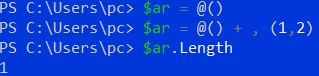# Create an Empty Array of Arrays in PowerShell

John Wachira Nov 15, 2022

This article demonstrates how we can create an empty array to store subarrays. We will start by discussing the basics of creating and assigning values to arrays and finally see how we can create empty arrays. Let’s jump right in.

## Create Arrays in PowerShell

There are two methods we can use to create arrays in PowerShell. We can directly assign some values to a variable and separate them using a comma.

Here is an example:

``````\$b = "Deji","KSI","Wassaabi","Dream","Jelly"
``````

Our variable `\$b` is now an array. We can access the values of our variable using the indexing method.

Our first value is stored in 0 while the second is stored in 1, and so on. Checkout the example below:

``````\$b
``````

This will return `Deji`, the first value of our array. We can also use negative indexing where `-1` is the last value, `-2` is the second last value, and so on.

``````\$b[-1]
``````

This should return `Jelly`, the last value in our array. Let’s confirm our case.We can also create Polymorphic arrays in PowerShell. These are simply arrays that contain different data types.

Below is an example of a Polymorphic array:

``````\$c = "Mike",'El',11,20.2
``````

We can also create empty arrays in PowerShell. We use such arrays when working with scripts.

We can assign values to the arrays later. Below is an example of an empty array:

``````\$d = @()
``````

If we check the length of the array, we should get zero.

``````\$d.Length
``````

We can assign values to the array as illustrated below:

``````\$d = @("Hi",1,30.6)
``````

## Create an Empty Array of Arrays in PowerShell

We have discussed how to create empty arrays and append values. However, creating an empty array to store subarrays follows a different process.

If we attempt to add a subarray to an array using the conventional method, PowerShell will concatenate the subarrays. Below is an example:

``````\$e = @()
\$e += @(1,2)
``````

If we check the length, it will give us 2 instead of 1.To add the subarray as a single element, we need to prefix the subarray to add with a comma, as illustrated below:

``````\$ar = @()
\$ar = @() + , (1,2)
``````

If we check the length, it should now be 1. Let’s confirm our case:In conclusion, there are various ways of creating arrays on powershell. We have discussed creating an empty array to store subarrays in PowerShell.

Author: John Wachira

John is a Git and PowerShell geek. He uses his expertise in the version control system to help businesses manage their source code. According to him, Shell scripting is the number one choice for automating the management of systems.